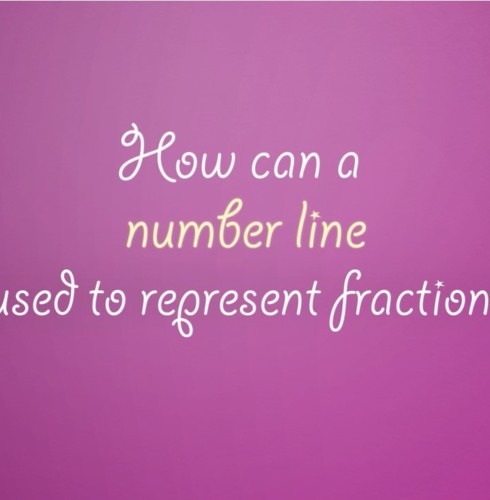## ArchivesIn 3rd grade, students are expected to generate simple equivalent fractions. Example: 1/2 = 4/8 and 4/6 = 2/3. Models should be used to help students identify equivalent fractions. Sentence strips or strips of paper cut into congruent rectangles can…In 3rd grade, students are expected to compare two fractions that have either the same numerator or the same denominator. When comparing fractions, it is important for students to understand the value of the fraction and consider both the numerator…For information on using an area model to represent fractions, visit: